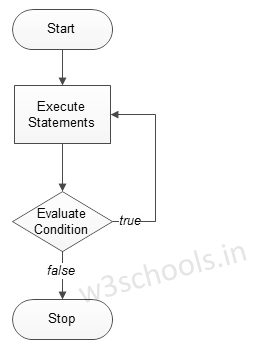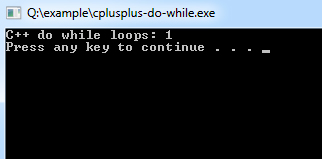## C++ Programming Tutorial Index

C++ `do while` loop is similar to the `while` loop, but it always executes a code block at least once and so on as long as the condition is `true`. This is an exit-controlled loop. This tutorial will teach you how to use the `do while` loop in C++.

The basic format of the `do while` loop statement is:

Syntax:

``````do
{
statement(s);
}
while( condition );``````

Figure - Flowchart of the `do while` loop:Example C++ program to demonstrate the `do while` loop:

Example:

``````#include <iostream>
using namespace std;

int main ()
{
/* local variable Initialization */
int n = 1,times=0;

/* do-while loops execution */
do
{
cout << "C++ do while loops: " << n <<endl;
n++;
}
while( n <= times );

return 0;
}``````

Program Output: